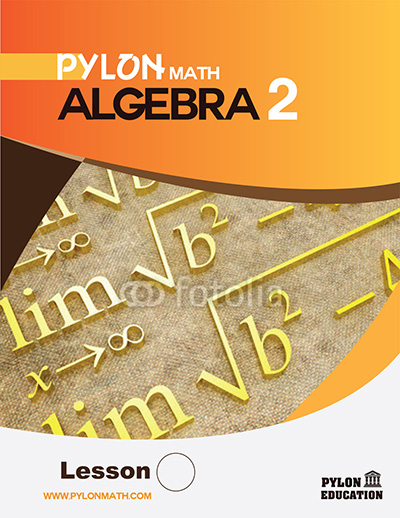# Algebra 21. ### Absolute Value Inequality

• Absolute Value Equations, Inequalities
• Graph, Set Notation, Interval Notation
• Real Numbers, Properties of Real Numbers
• Basic Skills
2. ### Functions

• Evaluation, Transformations, Parent Functions
• Review on Graphs, Absolute Value Functions
• Greatest Integer Functions
• Operations with Functions
• Composite Functions, Inverse
3. ### Graphs

• Systems of Three Equations
• Systems of Three Inequalities
• Linear Programing
• Linear Equations in Three Dimensions
4. ### Matrices

• Matrices and Data, Matrix Product
• Transformations, Reflection Matrix
• Rotation, Cramer’s Rule
• Solutions of Systems
• Determinant of 3x3 Matrix
• Inverses, Augmented Matrix

• Vertex Form, Transformations
• Completing the Square, Vertex Point
• Complex Numbers and Roots
• Sum and Product of Roots
• Curve Fitting with Quadratic Models
• Problem Solving, Complex Numbers
6. ### Polynomials

• Binomial Expression, Long Division
• Synthetic Division, Remainder Theorem
• Roots, Factoring, Multiplying of Roots
• Conjugate Root Theorem
• End Behavior
1. ### Logarithmic Functions

• Inverse Functions, Exponents
• Logarithmic Functions
• Natural Logarithms, Compound Interest
• Continuously Compound Interest
• Natural Decay, Half-Life
• Appreciation, Depreciation, Graphing
2. ### Rational Functions

• Direct, Indirect, Joint Variations
• Multiplying and Dividing Expressions
• Complex Fractions
• Vertical & Horizontal Asymptotes
• Domain, Range, Holes in Graphs
• Graphing Rational Functions
• Rational Equations and Inequalities
3. ### Irrational Numbers

• Graphs, Piecewise Functions
• Transforming Functions

• Circles
• Ellipses
• Hyperbolas
• Parabolas
5. ### Sequences

• Arithmetic Sequences
• Geometric Sequences
• Series, Infinite Geometric Series
• Recursive Sequences
6. ### Statistics

• Variability, Standard Value
• Normal Distribution
• Percentiles